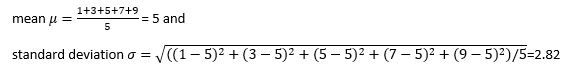top of pageSearch

# Feature ScalingFeature Scaling is a method to transform the numeric features in a dataset to a standard range so that the performance of the machine learning algorithm improves. It can be achieved by normalizing or standardizing the data values. This scaling is generally preformed in the data pre-processing step when working with machine learning algorithm.

Example, if we have weight of a person in a dataset with values in the range 15kg to 100kg, then feature scaling transforms all the values to the range 0 to 1 where 0 represents lowest weight and 1 represents highest weight instead of representing the weights in kgs.

In feature scaling, we scale the data to comparable ranges to get proper model and improve the learning of the model. The scale which we choose is not important but each of the feature in the dataset should be on the same scale. Example, if one feature is chosen to be in range 0 to 1 then all the remaining features in the same dataset should also be in range 0 to 1.

### Why Feature Scaling

If we consider a car dataset with below values:Here age of car is ranging from 5years to 20years, whereas Distance Travelled is from 10000km to 50000km. When we compare both the ranges, they are at very long distance from each other. The machine learning algorithm thinks that the feature with higher range values is most important while predicting the output and tends to ignore the feature with smaller range values. This approach would give wrong predictions.

To avoid such wrong predictions, the range of all features are scaled so that each feature contributes proportionately and model performance improves drastically.

Another reason for feature scaling is that if the values of a dataset are small then the model learns fast compared the unscaled data. Example, in gradient decent, to minimize the cost function, if the range of values is small then the algorithm converges much faster.

### Which Algorithms need Feature scaling?

The models which calculate some kind of distance as part of the algorithm needs the data to be scaled.

Example: Linear Regression, Logistic Regression, SVM, KNN, K-Means clustering, PCA etc.

### Which algorithms don’t need Feature scaling?

Tree based models where each node is split based on the condition doesn’t need the features to be scaled because the model accuracy don’t depend on the range. Moreover, if we scale the features here to the range 0 to 1 then many values are decimal values near to each other and constructing the tree takes more time.

Example: Decision Trees, Random Forest, XGBoost etc.

### Common Feature Scaling Methods

Normalization:

This is also known as Min-Max scaling. It scales the data to the range between 0 and 1. This scaling is performed based on the below formula.Where x is the current value to be scaled, min(X) is the minimum value in the list of values and max(X) is the maximum value in the list of values

Example: if X= [1,3,5,7,9] then min(X) = 1 and max(X) = 9 then scaled values would be:Here we can observe that the min(X) 1 is represented as 0 and max(X) 9 is represented as 1.

Python Implementation of Normalization:

Scikit-learn object MinMaxScaler is used to normalize the dataset. For this, first import the MinMaxScaler from sklearn and define an instance with default hyperparameters. Then call the fit_transform() function on the input data to create a transformed version of data.

```from sklearn.preprocessing import MinMaxScaler
trans = MinMaxScaler()
data_trans = trans.fit_transform(data)```

Standardization:

It represents the values in standard deviations from the mean.Where x is the current value to be scaled, µ is the mean of the list of values and σ is the standard deviation of the list of values.

The scaled values are distributed such that the mean of the values is 0 and the standard deviation is 1.

Example if X= [1,3,5,7,9] thenThen scaled values would be:Here the values are ranging from -1.41 to 1.41. This range changes depending on the values of X.

Python Implementation of Standardization:

Scikit-learn object StandardScaler is used to standardize the dataset. For this, first import the StandardScaler from sklearn and define an instance with default hyperparameters. Then call the fit_transform() function on the input data to create a transformed version of data.

```from sklearn.preprocessing import StandardScaler
scaled = StandardScaler()
data_scaled = scaled.fit_transform(data)```

### Normalization Vs Standardization

Standardization is useful when the values of the feature are normal distributed (i.e., the values follow the bell-shaped curve). Else (if vales are not normal distributed) Normalization is useful.

For complex models, which method performs well on an input data is unknown. In that case, model the data with standardization, Normalization and combination of both and compare the performances of resulting models.

Both the methods do not perform well when the values contain outliers. To fix this, prior check the out of bound values and change their values to the known minimum and maximum values.

### Summary

Feature scaling is achieved by normalizing or standardizing the data in the pre-processing step of machine learning algorithm. It improves the performance of the algorithm.